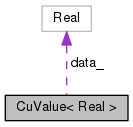CuValue< Real > Class Template Reference

The following class is used to simulate non-const references to Real, e.g. More...

`#include <cu-value.h>`

Collaboration diagram for CuValue< Real >:[legend]

## Public Member Functions

CuValue (Real *data)

CuValue (const CuValue &other)

CuValue operator= (const CuValue< Real > &other)

Real operator= (Real r)

Real operator+= (Real r)

Real operator-= (Real r)

operator Real () const

Real * data_

## Detailed Description

### template<typename Real> class kaldi::CuValue< Real >

The following class is used to simulate non-const references to Real, e.g.

as returned by the non-const operator (). This class is also used as a convenient way of reading a single Real value from the device.

Definition at line 34 of file cu-value.h.

## ◆ CuValue() [1/2]

 CuValue ( Real * data )
inline

Definition at line 36 of file cu-value.h.

36 : data_(data) { }
Real * data_
Definition: cu-value.h:86

## ◆ CuValue() [2/2]

 CuValue ( const CuValue< Real > & other )
inline

Definition at line 37 of file cu-value.h.

37 : data_(other.data_) { }
Real * data_
Definition: cu-value.h:86

## ◆ operator Real()

 operator Real ( ) const
inline

Definition at line 73 of file cu-value.h.

References CuValue< Real >::data_.

73  { // assignment to Real
74 #if HAVE_CUDA == 1
75  if (CuDevice::Instantiate().Enabled()) {
76  Real value;
77  CU_SAFE_CALL(cudaMemcpyAsync(&value, data_, sizeof(Real),
80  return value;
81  } else
82 #endif
83  return *data_;
84  }
Real * data_
Definition: cu-value.h:86

## ◆ operator+=()

 Real operator+= ( Real r )
inline

Definition at line 69 of file cu-value.h.

69 { return (*this = r + Real(*this)); }

## ◆ operator-=()

 Real operator-= ( Real r )
inline

Definition at line 70 of file cu-value.h.

70 { return (*this = Real(*this) - r); }

## ◆ operator=() [1/2]

 CuValue operator= ( const CuValue< Real > & other )
inline

Definition at line 39 of file cu-value.h.

References CuValue< Real >::data_.

39  {
40 #if HAVE_CUDA == 1
41  if (CuDevice::Instantiate().Enabled()) {
42  CU_SAFE_CALL(
43  cudaMemcpyAsync(data_, other.data_, sizeof(Real),
45  return *this;
46  } else
47 #endif
48  {
49  *data_ = *other.data_;
50  return *this;
51  }
52  }
Real * data_
Definition: cu-value.h:86

## ◆ operator=() [2/2]

 Real operator= ( Real r )
inline

Definition at line 54 of file cu-value.h.

References CuValue< Real >::data_.

54  { // assignment from Real
55 #if HAVE_CUDA == 1
56  if (CuDevice::Instantiate().Enabled()) {
57  CU_SAFE_CALL(cudaMemcpyAsync(data_, &r, sizeof(Real),
60  return r;
61  } else
62 #endif
63  {
64  *data_ = r;
65  return r;
66  }
67  }
Real * data_
Definition: cu-value.h:86

## ◆ data_

 Real* data_
private

Definition at line 86 of file cu-value.h.

Referenced by CuValue< Real >::operator Real(), and CuValue< Real >::operator=().

The documentation for this class was generated from the following file: